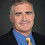## Friday, January 23, 2009

### Fooling Constant-Depth Circuits

Apparently Mark Braverman has settled a major open question about circuits due to Linial and Nisan. He shows that no poly-logarithmically independent distribution can be distinguished from a truly random distribution by a polynomial-size constant-depth circuit.

A r-independent distribution over binary strings of length n means that for every set of r bits are uniformly distributed over the 2r possible values for those bits. We can create r-independent distributions using O(r log n) truly random bits.

Braverman shows that no depth-d size-m circuit of AND, OR and NOT gates can distinguish between a truly uniform distribution and any r-independent distribution with r = (log m)O(d2).

In a celebrated 2007 FOCS paper, Bazzi proved the result for the special case for DNF formulas (depth-2 circuits). Razborov recently had a simpler proof of Bazzi's theorem.

Nisan already created specific pseudorandom generators for constant-depth circuits but Braverman's result allows us to use any poly-logarithmically independent set including some that come out of coding theory. While the result doesn't have immediately obvious applications, I expect this result to be a fundamental tool for complexity for years to come.

Scott has a longer take and somehow manages to connect it with quantum computing.

1.Actually the result requires r = (log m) to the O(d^2) independence for depth d.

2.Thanks. Fixed.

3.Should this result now be known as "Braverman's Theorem"?

4.Dear Lance, here is a problem (perhaps silly) that comes to mind: Is the conclusion of the Linial-Nisan conjecture (=Braverman’s result) holds for monotone threshold circuits, namely for bounded depth circuits with (monotone) threshold gates?

5.What are consequences and applications of this result?